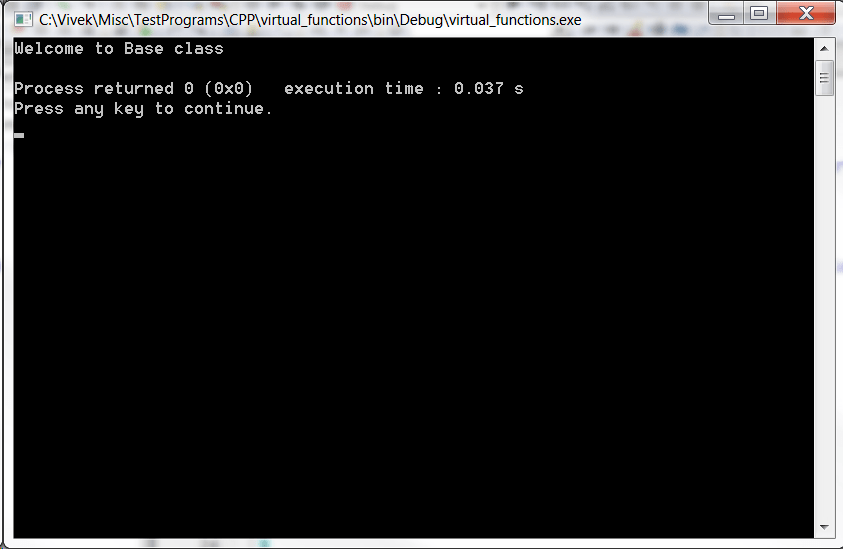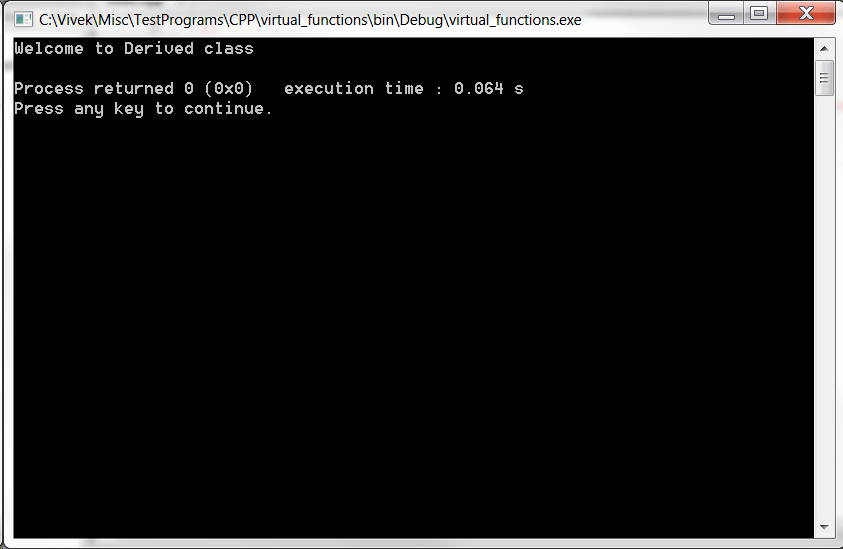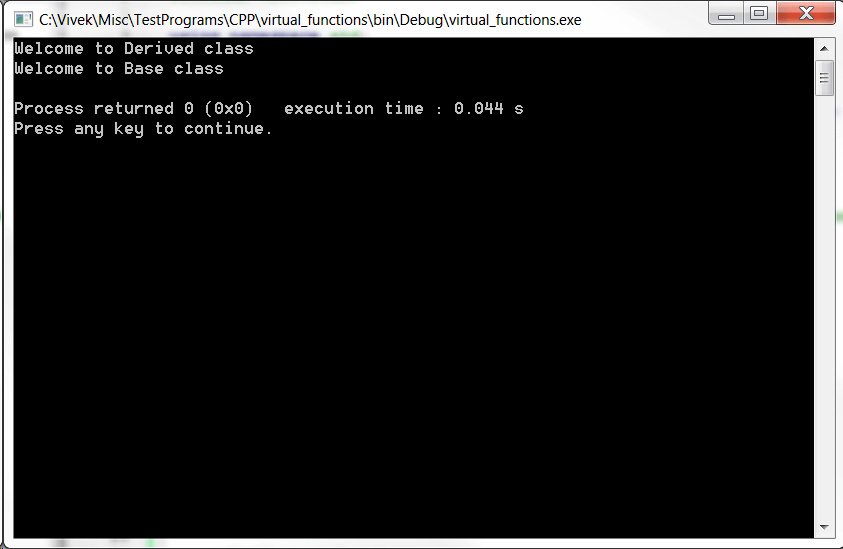Let’s look at the class definition below:

[code language=”cpp”]

class Base {
public:
void welcome_message() {cout << "Welcome to Base class" << endl;}
};

class Derived : public Base {
public:
void welcome_message() {cout << "Welcome to Derived class" << endl;}
};

[/code]

Lets assume we have to design a function which will take a pointer to the class object and print the welcome message by calling the class member function welcome_message(). Here is the function which can do the same for us:

[code language=”cpp”]

void printMessage(class Base *ptr)
{
ptr->welcome_message();
}

[/code]

Now, if we call the printMessage() with the Base class pointer it will print the message as below:

[code language=”cpp”]

int main()
{
Base *bptr = new Base();
printMessage(bptr);

return 0;
}

[/code]Now what about the Derived class, we would like to do the same with a Derived class pointer as well. Lets try in the same way as below:

[code language=”cpp”]

int main()
{
Derived *dptr = new Derived();
printMessage(dptr);

return 0;
}

[/code]

In the above program we have modified previously existing main() functionto create a Derived class object which is pointed by a Derived class type pointer dptr. Now, we pass the dptr pointer to printMessage() function and here is the output:As you can see the function printMessage() takes the dptr into a Base class type pointer ptr (look into the formal parameter in the function printMessage). Now, printMessage() doesn’t have any idea what is the object type and uses the pointer type to determine the object type which is Base class. Hence, it ends up calling the Base class version of the member function welcome_message(). This is certainly we didn’t want to do, what we wanted is that printMessage() would call the Derived class version of the welcome_message() but that didn’t happen. One solution to this problem is defining yet another printMessage() function with Derived class type pointer in the formal argument list, in other words overloading the printMessage() function as below:

[code language=”cpp”]

void printMessage(class Derived *ptr)
{
ptr->welcome_message();
}

[/code]

Now, if we execute the program again it will give us the desired output as below:However, this is not an optimized solution to the problem. We need common function/interfaces which can work for us both with a Base type object as well as with a Derived class object. And virtual function is what helps us achieving this. Lets see the redefined Base class definition as below which uses virtual keyword in front of the welcome_message() member function as below:

[code language=”cpp”]

class Base {
public:
virtual void welcome_message() {cout << "Welcome to Base class" << endl;}
};

class Derived : public Base {
public:
void welcome_message() {cout << "Welcome to Derived class" << endl;}
};

[/code]

Now, lets modify our main() function again to check if it has solved our problem yet:

[code language="cpp"]

int main()
{
Base *bptr = new Derived();

printMessage(bptr);

bptr = new Base();

printMessage(bptr);

return 0;
}

[/code]

Execute the code and the below output will be generated:As you can see the same pointer bptr first, points to a Derived class object, printMessage() is called with bptr and it prints the message “Welcome to Derived class”. Then the same bptr points to a Base class object and the printMessage() function prints the message “Welcome to Base class”.

So by using the virtual function mechanism we have been able to create a generic function called printMessage() which works for both the Base class object type as well as the Derived class object type.  These type of functions are called interfaces in c++.

Here is the full listing of the source code:

[code language=”cpp”]

#include

using namespace std;

class Base {
public:
virtual void welcome_message() {cout << "Welcome to Base class" << endl;}
};

class Derived : public Base {
public:
void welcome_message() {cout << "Welcome to Derived class" <welcome_message();
}

int main()
{
Base *bptr = new Derived();

printMessage(bptr);

bptr = new Base();

printMessage(bptr);

return 0;
}

[/code]

Note:

There is an inherent issue withe the above code and that is memory leak. As the bptr pointer has been overridden there is noway the Derived class object can be free. To get rid of this should call a delete() on bptr before overriding it with the Base class object as below:

[code language=”cpp”]

int main()
{
Base *bptr = new Derived();

printMessage(bptr);

delete(bptr);

bptr = new Base();

printMessage(bptr);

return 0;
}

[/code]